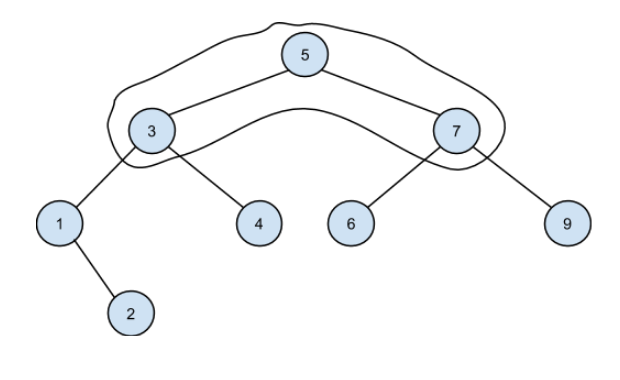New update is available. Click here to update.
Topics

# Max element in the path

Easy0/40
Average time to solve is 15m## Problem statement

Given a Binary Search Tree and two integers NODE1 and NODE2. You have to find the maximum element in the path from NODE1 to NODE2.

A binary search tree (BST), also called an ordered or sorted binary tree, is a rooted binary tree whose internal nodes each store a key greater than all the keys in the node's left subtree and less than those in its right subtree.

Note:

``````1. The path from NODE1 to NODE2 does not include NODE1 and NODE2.

2. NODE1 and NODE2 are unique.

3. If there is no element in the path from NODE1 to NODE2, return -1.
``````
Detailed explanation ( Input/output format, Notes, Images )
Constraints
``````1 <= T <= 100
1 <= N <= 3000
0 <= DATA <= 10 ^ 9
1 <= NODE1, NODE2 <= 10 ^ 9

Where ‘T’ is the total number of test cases, 'N' denotes the number of nodes in the given BST,  'DATA' denotes the value of each node in the given tree, and NODE1 and NODE2 are 2 integers.

Time limit: 1 sec.
``````
Sample Input 1:
``````1
5 3 7 1 4 6 9 -1 2 -1 -1 -1 -1 -1 -1 -1 -1
1 9
``````
Sample Output 1:
``````7
``````
Explanation of sample input 1:
``````The BST of the given elements is shown below.
````````````The path from 1 to 9 is shown in the curve.
As we can see, the maximum element in the path from 1 to 9 is 7.
``````
Sample Input 2:
``````2
2 1 4 -1 -1 3 -1 -1 -1
3 5
7 5 8 -1 6 -1 10 -1 -1 9 -1 -1 -1
6 7
``````
Sample Output 2:
``````-1
5
``````
Explanation of sample input 2:
``````Test case 1:
As 5 does not exist in the BST, simply return -1.

Test case 2:
After creating the BST for the given level order, we can see that the 5 is the maximum element between 6 and 7.
``````Console